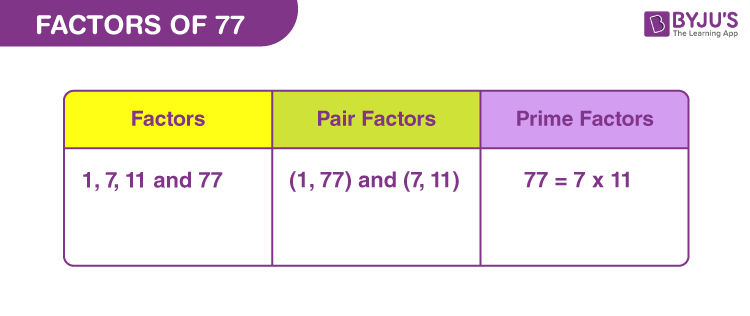# Factors of 77

Factors of 77 are the natural numbers that can exactly divide the original number. If the product of two numbers is equal to 77, then the numbers are the required factors.

If m x n = 77, then m and n are the factors.

Also,

• 77/m = n
• 77/n = m

Therefore, the factors of 77 are also called divisors.

Let us find the factors, pair factors and prime factors of 77 here in this article.## How to Find the Factors of 77?

The factors of 77 can be determined easily by the division method. Also, another way is to find the product of two integers that will produce 77. Since 77 is a composite number, it will have more than two factors. Let us find these factors here.

77 ÷ 1 = 77

77 ÷ 7 = 11

77 ÷ 11 = 7

77 ÷ 77 = 1

Therefore, the factors of 77 are 1, 7, 11 and 77.

 Facts: 77 is an odd number. It cannot be divided by 2 1 is the factor of all the numbers, hence 77 also 77 is the factor of itself 77 is a composite number

## Pair Factors of 77

Pair factors or factors in pairs are evaluated when the multiplication of two numbers results in the original number.

• 1 × 77 = 77
• 7 × 11 = 77

Therefore, the pair factors of 77 are (1, 77) and (7, 11).

If we consider the negative factors in pairs to get 77, then;

• -1 × -77 = 77
• -7 × -11 = 77

Therefore, the negative pair factors are (-1, -77) and (-7, -11).

## Prime Factorisation of 77

By prime factorisation method, we can evaluate the prime factors of 77, that can divide the original number evenly.

 Dividing 77 by smallest prime number, i.e., 7 77 ÷ 7 = 11 11 is a prime number and is divisible by 11 11 ÷ 11 = 1

Therefore, the prime factors of 77 are 7 and 11.

 Prime factorisation of 77 = 7 x 11 Exponential form = 71 x 111

Also check: Prime Numbers

## Solved Examples

Q.1: Two ribbons are 77 cm and 88 cm long. Roohi wants to cut the ribbons into pieces of equal length. What will be the maximum length of each piece of ribbon?

Solution: Given,

Length of ribbons are 77 cm and 88 cm, respectively.

To find the maximum length of the ribbon, we have to find the greatest common factor (GCF) of both the given lengths, 77 and 88. Thus,

77 → 1, 7, 11 and 77

88 → 1, 2, 4, 8, 11, 22, 44, 88

GCF of 77 and 88 is 11

Therefore, the maximum length of each piece of ribbon is 11 cm.

Q.2: What is the sum of all the factors of 77?

Solution: The factors of 77 are 1, 7, 11 and 77.

Sum = 1 + 7 + 11 + 77 = 96

Therefore, the required sum is 96

Q.3: What are the common factors of 77 and 87?

Solution: We have to list the factors of 77 and 87.

77 → 1, 7, 11, 77

87 → 1, 3, 29, 87

Therefore, the common factor of 77 and 87 is 1.

Q.4: What is the highest common factor of 77 and 56?

Solution: Factors of the numbers are:

56 → 1, 2, 4, 7, 8, 14, 28, 56

77 → 1, 7, 11, 77

Highest common factor of 56 and 77 is 7.

### Practice Questions

1. Calculate the average of factors of 77.
2. What are the divisors of 77?
3. Find if 17 is the factor of 77.
4. Find the greatest factor that divides both 77 and 99.

## Frequently Asked Questions on Factors of 77

### What are the factors of 77?

The factors of 77 are 1, 7, 11 and 77.

### What are the multiples of 77?

The first ten multiples of 77 are 77, 154, 231, 308, 385, 462, 539, 616, 693 and 770.

### What is the prime factor form of 77?

The prime factorisation of 77 is 7 x 11.

### Which is the second highest factor of 77?

The second highest factor of 77 is 11.

### Is 77 a perfect square?

77 is not a perfect square, because the square root of 77 is a fraction.